SEARCH HOMEMath Central Quandaries & QueriesSubject: cross multiplication Name: Kayla Who are you: Other Why does eight over twelve compared to one half work when you use cross multiplication.Hi Kayla,

I am going to look at two different fractions. Suppose you want to compare 2/3 and 5/8 to see which is larger. It would be easy to compare them if they had the same denominator so I am going to use a common denominator (in this case 35 = 24).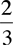=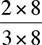=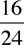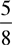=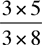=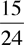Since 16 > 15 you can conclude that>Now look at the second fractions in the two lines above,and. The denominators are equal and the numerators are the result of cross multiplying 2/3 an 5/8.

I hope this helps,
PennyMath Central is supported by the University of Regina and The Pacific Institute for the Mathematical Sciences.